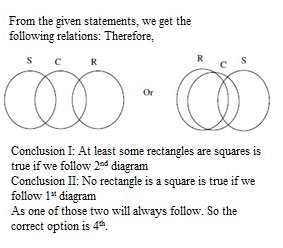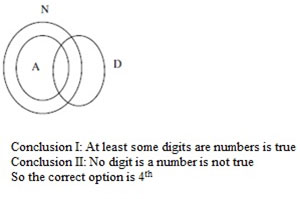# Mu Sigma Reasoning Question

DIRECTIONS for the questions 1 to 2 :The question below has either two or three statements followed by two or three conclusions. You have to take the given statements to be tdue and then decide which of the given conclusions logically follows from the given statements, disregarding the commonly known facts.

1. Statement: Some squares are circles, Some circles are rectangles.
Conclusion: I.At least some rectangles are squares.
II.No rectangle is a square.
1. Only conclusion II follows
2. Only conclusion I follow
3. Neither conclusion I nor II follows
4. Either conclusion I or II follows
5. Both conclusions I and II follow1. Statement: Some squares are circles, Some circles are rectangles.
Conclusion: I.At least some rectangles are squares.
II.No rectangle is a square.
1. Only conclusion II follows
2. Only conclusion I follow
3. Neither conclusion I nor II follows
4. Either conclusion I or II follows
5. Both conclusions I and II followDIRECTIONS for the questions 3 to 5:Read the information given below and answer the question that follows.
A, B, C, D, E, F and G are standing in a stdaight line facing north with equal distance between them, not necessary in the same order. Each one is pursuing a different profession - actor, reporter, doctor, engineer, lawyer, teacher and painter not necessary in the same order. G is fifth to the left of C. The reporter is third to the right of G. F is fifth to the right of A. E is second to the left of B. The engineer is second to the left of D. There are only three people between the engineer and the painter. The doctor is to the immediate left of the engineer. The lawyer is to the immediate right of the teacher.
2. Who amongst the following is the group of engineer and teacher?
1. BG
2. FE
3. GE
4. BA
5. CG
From the given information, we have the following arrangement:
 A G E D B F C Doctor Engg Teacher Lawyer Reporter Painter Actor
G is the engineer and E is the teacher. Hence option C.
3. What is D's position with respect to the painter?
1. Third to the left
2. Second to the right
3. Fourth to the right
4. Third to the right
5. Second to the left
From the given information, we have the following arrangement:
 A G E D B F C Doctor Engg Teacher Lawyer Reporter Painter Actor
D's position with respect to the painter is second to the left. Hence option E.
4. Who among the following are standing at the extremes ?
1. A and F
2. G and C
3. G and F
4. A and B
5. A and C
 A G E D B F C Doctor Engg Teacher Lawyer Reporter Painter Actor
A and C are standing at the extdemes. Hence option E.
DIRECTIONS for the questions 6 : Solve the following question and mark the best possible option.
In a certain code,
'very large risk associated' is written as 'nu ta ro gi'
'risk is very low' is written as ' gi se nu mi'
'is that also associated' is written as 'ta mi po fu'
'inherent risk also damaging' is written as 'fu nu di yu'
(All the codes are two letter codes only.)
5. What is the code for 'very'?
1. ta
2. fu
3. ro
4. nu
5. Gi
'very large risk associated' is written as 'nu ta ro gi'-(1)
'risk is very low' is written as ' gi se nu mi-----(2)
'is that also associated' is written as 'ta mi po fu'----(3)
'inherent risk also damaging' is written as 'fu nu di yu'---(4)
From statements 1 and 3, we have 'associated' as the common word and the common code is 'ta'
From statements 3 and 4, we have 'also' as the common word and the common code is 'fu'
From statements 2 and 4, we have 'risk' as the common word and the common code is 'nu
' From statements 2 and 3, we have 'is' as the common word and the common code is 'mi'
From statements 1 and 2, we can conclude that the code of 'very' is 'gi'
Therefore, from statement 1, the code of 'large' will be 'ro'
Similarly, from statement 2, the code of 'low' is 'se' and from statement 3, the code of 'that' is 'po'
 Word Code Very Gi risk Nu Is Mi Also Fu Low Se large ro That Po Associated Ta inherent Di/yu damaging Di/yu
The code for 'very' is 'gi'. Hence option E
DIRECTIONS for the questions 7: Solve the following question and mark the best possible option.
Point A is 14m north of point B
Point C is 11m east of point B
Point D is 5m north of point C
Point E is 7m west of point D
Point F is 6m north of point E
Point G is 4m west of point F
6. How for and in which direction is point G from point A?
1. 3m north
2. 5m north
3. 4m north
4. 4m south
5. 3m south
G is 3m south from A. Hence option 5DIRECTIONS for the questions 8 : The question below consists of a question and two statements numbered I and II given below it. You have to decide whether the data provided in the statements are sufficient to answer the question. Read both the statements and mark
7. I. C is second to the left of A, B and D are immediate neighbors of each other
II. D is the immediate left of B. E is not an immediate neighbor of D and B
What is E's position with respect to D?
1. The data in both the Statements I and II together are necessary to answer the question
2. The data either in Statement I alone or in Statement II alone are sufficient to answer the question
3. The data in Statement I alone are sufficient to answer the question, while the data in Statement II alone are not sufficient to answer the question
4. The data in Statement II alone are sufficient to answer the question, while the data in Statement I alone are not sufficient to answer the question
5. The data neither in Statement I nor in Statement II are sufficient to answer the question8. Among five friends - J, K, L, M and N each of a different height who is the second tallest
I. N is taller than M and K, K is shorter than M.
II. L is taller than N. J is not the tallest
.
1. The data in Statement II alone are sufficient to answer the question, while the data in Statement I alone are not sufficient to answer the question
2. The data neither in Statement I nor in Statement II are sufficient to answer the question
3. The data in both the Statements I and II together are necessary to answer the question
4. The data in Statement I alone are sufficient to answer the question, while the data in Statement II alone are not sufficient to answer the question
5. The data either in Statement I alone or in Statement II alone are sufficient to answer the question
From statement I, we get N>M>K but we don't know the position of J and L. So we can't figure out who is the 2nd tallest From statement II, we get L>N and J is not the tallest but again we can't say anything about the 2nd tallest person On combining both the statements, we get L>N>M>K only and J can be anywhere in between L and K. So we can't say anything who is the 2nd tallest person Therefore, the data neither in Statement I nor in Statement II are sufficient to answer the question. Hence option B.
DIRECTIONS for the questions 10 : Solve the following question and mark the best possible option.
9. Statement: J = A; C ≥ K > S ≥ A
Conclusion: I. C > J II. C = J.
1. Neither conclusion I nor II follows
2. Both conclusions I and II follows
3. Only conclusion I follows
4. Only conclusion II follows
5. Either conclusion I or II follows